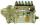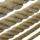A sculptor

A sculptor is duplicating a statue based on an original statue. If the scale factor of the replication is 3.2, will the new statue be larger or smaller than the original statue (enter 1 = larger, 0= smaller)

Result

x =  1

Solution:Leave us a comment of this math problem and its solution (i.e. if it is still somewhat unclear...):Be the first to comment!To solve this verbal math problem are needed these knowledge from mathematics:

Do you want to convert length units? Tip: Our volume units converter will help you with the conversion of volume units.

Next similar math problems:

1. Scale of the mapDetermine the scale of the map if the actual distance between A and B is 720 km and distance on the map is 20 cm.
2. Feet to milesA student runs 2640 feet. If the student runs an additional 7920 feet, how many total miles does the student run?
3. TreesA young tree is 16 inches tall. One year later, it is 20 inches tall. What is the percent increase in height?
4. DonutsFind how many donuts each student will receive if you share 126 donuts in a ratio of 1:5:8
5. Masons1 mason casts 30.8 meters square in 8 hours. How long casts 4 masons 178 meters square?
6. Car consumptionThe car has consumption 4 l/100 km. How much money will it cost ride 4 km in long if diesel price is 1.53 USD/l?
7. ClassIn a class are 32 pupils. Of these are 8 boys. What percentage of girls are in the class?
8. NumberWhat number is 20 % smaller than the number 198?
9. Liters od milkThe cylinder-shaped container contains 80 liters of milk. Milk level is 45 cm. How much milk will in the container, if level raise to height 72 cm?
10. TVsProduction of television sets increased from 3,500 units to 4,200 units. Calculate the percentage of production increase.
11. StringDivide the string length 800 cm in ratio 1: 5
12. Percents - easyHow many percent is 432 out of 434?
13. Simplify 2Simplify expression: 5ab-7+3ba-9
14. Base, percents, valueBase is 344084 which is 100 %. How many percent is 384177?
15. InequationSolve the inequation: 5k - (7k - 1)≤ 2/5 . (5-k)-2
16. Unknown numberIdentify unknown number which 1/5 is 40 greater than one tenth of that number.
17. EquationSolve the equation: 1/2-2/8 = 1/10; Write the result as a decimal number.